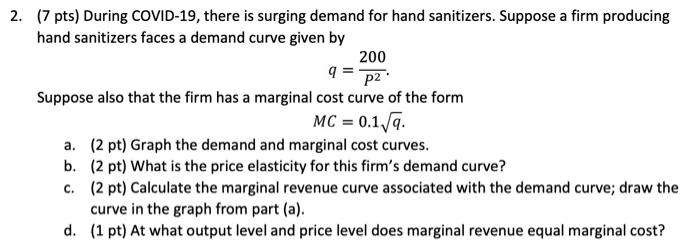# Question Solved1 Answer2. (7 pts) During COVID-19, there is surging demand for hand sanitizers. Suppose a firm producing hand sanitizers faces a demand curve given by 200 q = P2 Suppose also that the firm has a marginal cost curve of the form MC = 0.179. a. (2 pt) Graph the demand and marginal cost curves. b. (2 pt) What is the price elasticity for this firm's demand curve? C. (2 pt) Calculate the marginal revenue curve associated with the demand curve; draw the curve in the graph from part (a). d. (1 pt) At what output level and price level does marginal revenue equal marginal cost?Transcribed Image Text: 2. (7 pts) During COVID-19, there is surging demand for hand sanitizers. Suppose a firm producing hand sanitizers faces a demand curve given by 200 q = P2 Suppose also that the firm has a marginal cost curve of the form MC = 0.179. a. (2 pt) Graph the demand and marginal cost curves. b. (2 pt) What is the price elasticity for this firm's demand curve? C. (2 pt) Calculate the marginal revenue curve associated with the demand curve; draw the curve in the graph from part (a). d. (1 pt) At what output level and price level does marginal revenue equal marginal cost?
More
Transcribed Image Text: 2. (7 pts) During COVID-19, there is surging demand for hand sanitizers. Suppose a firm producing hand sanitizers faces a demand curve given by 200 q = P2 Suppose also that the firm has a marginal cost curve of the form MC = 0.179. a. (2 pt) Graph the demand and marginal cost curves. b. (2 pt) What is the price elasticity for this firm's demand curve? C. (2 pt) Calculate the marginal revenue curve associated with the demand curve; draw the curve in the graph from part (a). d. (1 pt) At what output level and price level does marginal revenue equal marginal cost?